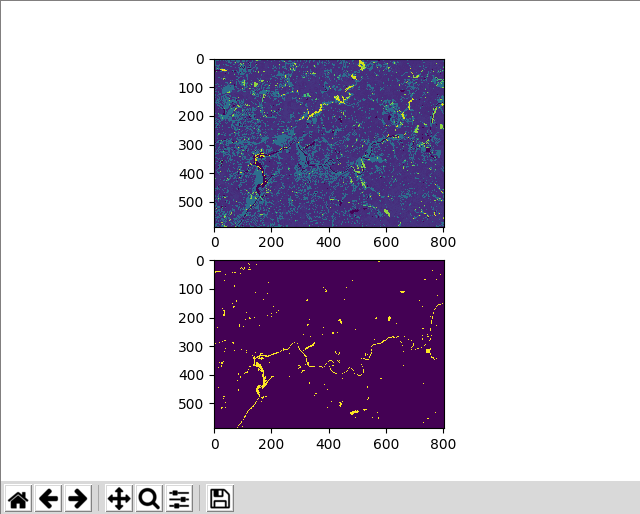# How to calculate the area of a certain class in a GeoTIFF file using NumPy

Institute for Environmental and Spatial Analysis...University of North Georgia

## 1   Introduction

Try these exercises first:

In this exercise, we want to calculate the area of a certain class in a GeoTIFF file. Let’s calculate the area of waterbodies in an NLCD dataset. Use nlcd2016_clipped.tif.## 2   Python code

``````from osgeo import gdal
import numpy as np
import matplotlib.pyplot as plt

ds = gdal.Open(filename)
band = ds.GetRasterBand(1)
return arr, ds

def write_geotiff(filename, arr, in_ds):
if arr.dtype == np.float32:
arr_type = gdal.GDT_Float32
else:
arr_type = gdal.GDT_Int32

driver = gdal.GetDriverByName("GTiff")
out_ds = driver.Create(filename, arr.shape, arr.shape, 1, arr_type)
out_ds.SetProjection(in_ds.GetProjection())
out_ds.SetGeoTransform(in_ds.GetGeoTransform())
band = out_ds.GetRasterBand(1)
band.WriteArray(arr)
band.FlushCache()
band.ComputeStatistics(False)

nlcd16_water_arr = np.where(nlcd16_arr == 11, 1, 0)
nlcd16_water_ncells = np.sum(nlcd16_arr == 11)

# https://gdal.org/tutorials/geotransforms_tut.html
dx = nlcd16_ds.GetGeoTransform()
dy = -nlcd16_ds.GetGeoTransform()

print("Total area of waterbodies is", nlcd16_water_ncells * dx * dy)

write_geotiff("nlcd16_water.tif", nlcd16_water_arr, nlcd16_ds)

plt.subplot(211)
plt.imshow(nlcd16_arr)

plt.subplot(212)
plt.imshow(nlcd16_water_arr)

plt.show()``````

## 3   Explanation

Import necessary modules and define the read and write functions.

``````from osgeo import gdal
import numpy as np
import matplotlib.pyplot as plt

ds = gdal.Open(filename)
band = ds.GetRasterBand(1)
return arr, ds

def write_geotiff(filename, arr, in_ds):
if arr.dtype == np.float32:
arr_type = gdal.GDT_Float32
else:
arr_type = gdal.GDT_Int32

driver = gdal.GetDriverByName("GTiff")
out_ds = driver.Create(filename, arr.shape, arr.shape, 1, arr_type)
out_ds.SetProjection(in_ds.GetProjection())
out_ds.SetGeoTransform(in_ds.GetGeoTransform())
band = out_ds.GetRasterBand(1)
band.WriteArray(arr)
band.FlushCache()
band.ComputeStatistics(False)``````

Read the data into a NumPy array and get the GDAL dataset of the file. Remember, `read_geotiff()` returns two items.

``nlcd16_arr, nlcd16_ds = read_geotiff("nlcd2016_clipped.tif")``

Based on the NLCD classification table, we extract open water cells only using the `nlcd16_arr == 11` condition where 11 indicates the class of open water.

``nlcd16_water_arr = np.where(nlcd16_arr == 11, 1, 0)``

Using the same condition `nlcd16_arr == 11`, count the number of open water cells. If a cell value is equal to 11, its value in the boolean array `nlcd16_arr == 11` is `True`. If not, its value is `False`. The shape of this boolean array is the same as that of the original `nlcd16_arr` array. Now, using `np.sum()`, we add all the cell values in the boolean array. For this summation, `True` is equivalent to 1 and `False` to 0. In other words, we add 1 as many times as there are `True` cells and 0 as many times as there are `False` cells. However, since 0 does not really contribute to the summation result and 1 only contributes to it once, the result of this summation is the number of `True` cells. Which cells are `True` again? Yes, open water cells. Finally, we have the number of open water cells in the variable `nlcd16_water_ncells`.

``nlcd16_water_ncells = np.sum(nlcd16_arr == 11)``

By referring to the geotransform tutorial, we extract the resolution of the NLCD dataset using the `GetGeoTransform()` function in the input dataset (`nlcd16_ds`). At index 1, we can find the x-resolution. The y-resolution is at index 5, but it is negative for a north-up image and we need to invert that (`-nlcd16_ds.GetGeoTransform()` instead of just `nlcd16_ds.GetGeoTransform()`).

``````# https://gdal.org/tutorials/geotransforms_tut.html
dx = nlcd16_ds.GetGeoTransform()
dy = -nlcd16_ds.GetGeoTransform()``````

The area of each cell can be calculated by multiplying the x- and y-resolutions. Then, the total area of waterbodies is calculated by multiplying the number of waterbody cells and the cell area.

``print("Total area of waterbodies is", nlcd16_water_ncells * dx * dy)``

Save the waterbodies array.

``write_geotiff("nlcd16_water.tif", nlcd16_water_arr, nlcd16_ds)``

Finally, plot all the arrays.

``````plt.subplot(211)
plt.imshow(nlcd16_arr)

plt.subplot(212)
plt.imshow(nlcd16_water_arr)

plt.show()``````